# Chapter 3b: cycloalkanes

Cycloalkanes

A cycloalkane is, as it name shows, a cyclic alkane chain. Each carbon of the chain is bound to (at least) two carbons and two hydrogen’s. The general formula is thus CnH2n and the name of the compound is the same name as the corresponding alkane with the prefix cyclo.

Cyclopropane

The smallest cycle, cyclopropane, is made of 3 carbons. Each carbon is bound to the two others with a triangle shape.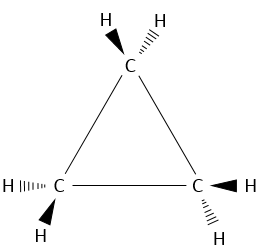That means that the carbons are in the same plane and that the angle between liaisons is 60°. This angle is far from the normal angle between liaisons in alkanes. Remember that carbons have a tetrahedral structure with an angle of 109.5° between each liaison. To be cyclic, there is a deformation of the carbon structure and a tension of cycle is maintained. It is possible to determine the importance of this tension from the energy of combustion ΔH°comb of the cycloalkane.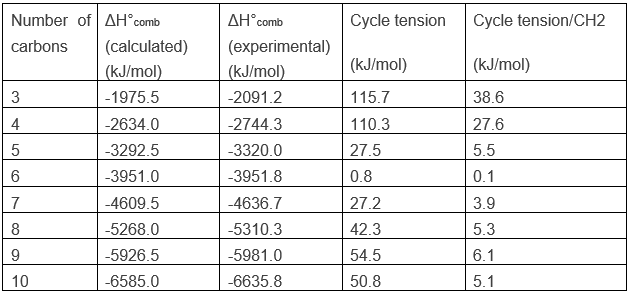For a linear alkane, the heat of combustion increases by approximately 658.5kJ/mol when the length of the chain is increases by one unit. One can conclude that the average ΔH°comb of a CH2 is 658.5kJ/mol. Applying this to the cyclopropane, C3H6, the calculated ΔH°comb is -1975.5kJ/mol. However, when we experimentally perform the combustion, we find that ΔH°comb =-2091.2kJ/mol.

The cyclopropane releases thus more heat than what we could expect. The difference, 115.7kJ/mol (38.6kJ/mol/CH2), comes from the cycle tension, i.e. the molecule requires more energy just to bind this way. In fact, the orbitals of the carbon are not well aligned but the angle between orbitals is 104°.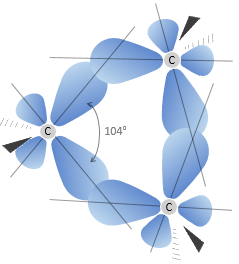As a result, the liaisons are weak and cyclopropane is not very stable. It is indeed easily open through catalytic hydrogenation.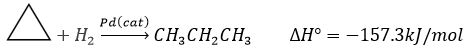Finally, the position of the hydrogen atoms is unfavourable. Let’s have a quick reminder at the eclipsed and staggered conformations for the hydrogen’s in ethane (C2H6). Hydrogen’s can rotate around the axe formed by the liaison C-C. Each hydrogen has a given volume and feels the atoms in its vicinity (steric hindrance). When rotating, for a given H the distance with the closest hydrogen carried by the other carbon changes.

On the Newman projection,

• the hydrogen’s are on the same spots, or so called eclipsed
• the hydrogen’s are not on the same spots, or so called staggered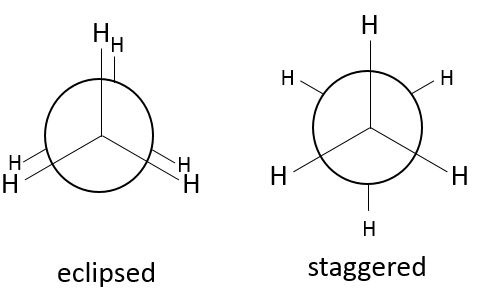A maximum of energy is reached in the eclipse conformation because repulsion between the hydrogen’s is maximal in this conformation. Cyclopropane has to maintain its hydrogen’s (or its substituents) eclipsed and it requires a lot of energy to the molecule. In the cyclopropane, all the hydrogen’s are eclipsed. The difference of energy between the eclipsed and the staggered conformations can be significant as we will see for cycles of more than 3 carbons.

Cyclobutane

Performing the combustion experiment on cyclobutane, for which the angles are of ~90°, we find an excess of 110.3kJ/mol (27.6kJ/mol/CH2) due to the cycle tension. It is less than for the cyclopropane because the forced bending is smaller in the cyclobutane than in the cyclopropane. The tension for these two cycles are very important. For larger cycles the tension decreases significantly and is at its minimum for a cycle of 6 carbons, the cyclohexane.

Cycles with more than 3 carbons are not plane. In the cyclobutane, the angle between the fourth carbon of the molecule, out of the plane, and the plane formed by the three other carbons is 26°.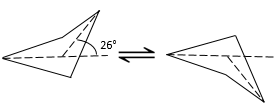The angle between the carbons is 88.5°. It is slightly less than for a square (90°) in one single plane. So why is the cyclobutane not plane? It should indeed decrease the cycle tension. However, in the plane conformation the 8 Hydrogen’s would be eclipsed. The 26° shaping of the cyclobutane moves the Hydrogen’s out of the eclipsed conformation. The small angle difference is thus counterbalanced by the improvement of the positioning of the Hydrogen’s.

The structure of the cyclobutane is oscillating quickly between two conformers: the carbon out of the plane moves from one side of the plane to the other. These two conformations are equivalent in energy. Looking at the next figure, 4 H are represented.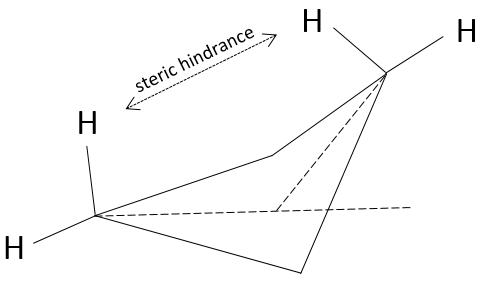Two of them (going upwards) are pretty close from each other while the two other are distant.  After the oscillation, the roles are inverted and the two Hydrogen’s have in average the same steric hindrance, also called transannular tension in this case.

Cycles can thus oscillate between several conformations when the conformations have a similar potential energy and if the energetic barrier between to switch of conformation is small enough. In the case of the cyclobutane the hindrance is very small, but if one of those 4 hydrogen’s was a substituent, the two conformers are no more equivalent. Indeed, the molecule will place itself  in the most favorable conformation which is when the voluminous substituent is not affected by the transannular tension. The proportion of the conformers is no more 50:50.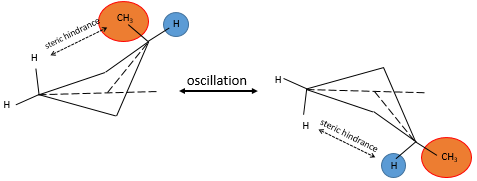Cyclopentane

The disfavour of the Hydrogen’s in ecliptic conformations is clear in the pentane. In a regular pentagon, the angle is 108°. It is almost the normal angle for a tetrahedral carbon (109.5°). However, 10 Hydrogen’s would be eclipsing one each other. The cyclopentane, and in fact any cycloalkane except the cyclopropane, is not plane. Two conformations (each with two conformers) are possible: the envelope and the semi-chair.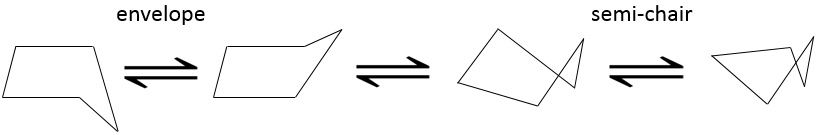In the envelop conformation, 4 carbons are in the same plane, with an angle of 104.4°.  In the other conformation (semi-chair), the angles are smaller but the eclipsing effect is smaller as well. The two conformations have very near potential energies and the barriers to switch between two forms are easily passed through. Cyclopentane oscillates then quickly between its conformers.

Cyclohexane

The case of the cyclohexane is particular. When we look at the heat of combustion of this species, the experimental value is less than one (0.8) kJ/mol different from the calculated one based on the number of CH2 in the molecule. Cyclohexane is the most stable existing cycloalkane.

Two conformations exist but one is more stable than the other one.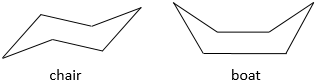The most stable conformation is the chair conformation.

In this conformation, 2 pairs of carbons are in the same plane and the last 2 carbons are in each side of the plane.  This position is called chair: the 4 carbons on the plane make the seat, one plane of 3 carbon makes the back of the chair and the other makes the footrest.

The angle between carbons is 111.4°, i.e. almost the 109.5° of a normal tetrahedral carbon, and all the Hydrogen atoms are in a staggered conformation.

This structure is thus very stable. Two types of Hydrogen’s can be distinguished: the ones in the axial position and the ones in the equatorial position. 6 liaisons C-H are parallel to the axe of the molecule (axe passing in the middle of the molecule). These are the axial Hydrogen’s. The other 6 liaisons are almost perpendicular and are called equatorial.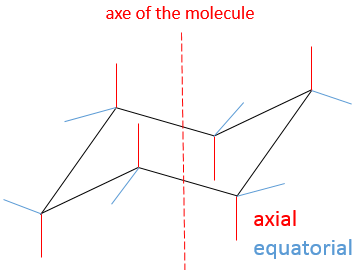In we reverse the chair structure (chair’s back<->footrest), equatorial hydrogen’s become the axial ones and vice versa.

To reverse the chair, cyclohexane has to go through the boat conformation, less stable by 28.9kJ/mol (the energetic barrier is 45.2kJ/mol). In this conformation, the two carbons that were out of the plane are now at the same side of it. This does not only generate a steric hindrance, but the hydrogen’s on the 4 carbons of the plane are now eclipsing each other. That explain the difference in potential energy between the boat and the chair formations.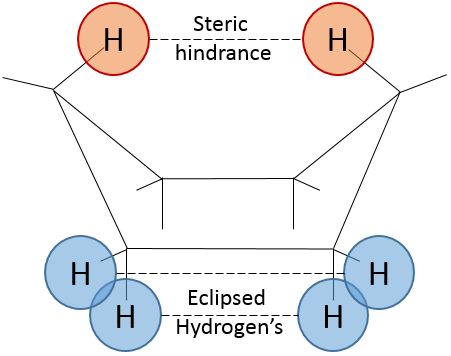In reality, this conformation is only a transition state. A more stable form is the crossed-boat conformation, almost identical but reducing the transannular tension. The boat conformation is thus the transition state between the two crossed-boat conformations.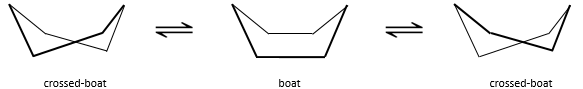We can resume the conformations as follow: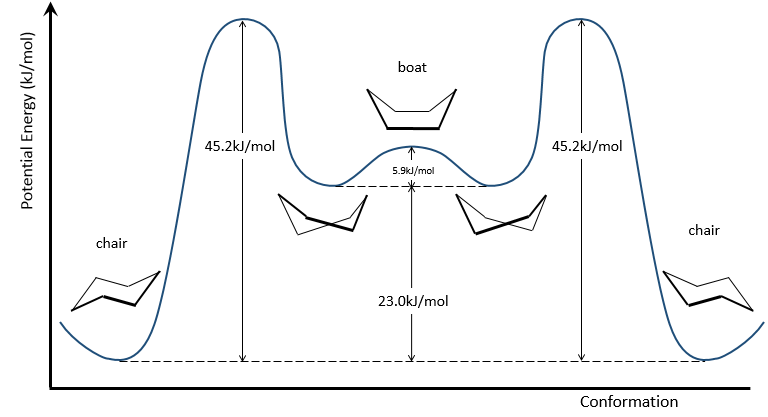Cyclohexane in the boat conformations only exist in very small proportions with regard to the chair conformation. We will thus only focus on the chair conformation for our further analyses.

Presence of substituents on the cyclohexane

The positions of a substituent on the cyclohexane are not equivalent.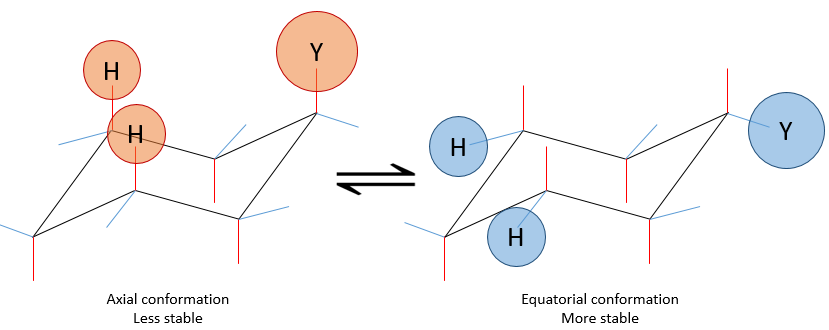If a substituent is placed on an axial position, the steric hindrance is greater than on an equatorial position. Indeed, equatorial substituents are more spaced than axial ones which go in the same direction. This is called the 1,3-diaxial interaction. The equatorial position for a substituent is then more stable than the axial one and one conformation of the molecule is favoured. For example, if the substituent is a methyl, the difference of energy between the conformations is 7.1kJ/mol, leading to a proportion of 95:5 (equatorial/axial).  Larger substituents increase furthermore the proportion of conformers.

The Newman projection can help to visualise this phenomenon. The whole molecule is represented, connecting two Newman projections together.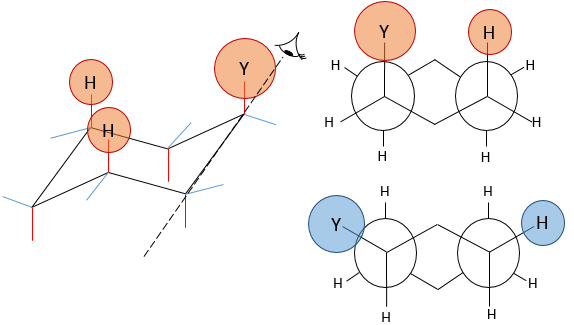Several substituents may be bound to a cyclohexane. The influence on the stability of each one is, for most of the substituents, simply additive. In the case of two substituents, one will be placed in an equatorial position anyway because it requires less energy than on an equatorial spot (as we just saw for the case of a single substituent). This substituent is the biggest one to minimize the steric hindrance. The second one, smaller, is either on an axial or an equatorial spot, depending on the connectivity of the molecule. If both groups are in equatorial positions, the equilibrium is further balanced towards this conformation. If the second substituent is on an axial spot, the equilibrium moves toward the 50:50 composition, the effects of the substituents acting against one each other.

Let’s see it through some examples. As explained before, the presence of a methyl on the cyclohexane favours the equatorial conformation by 7.1 kJ/mol. The presence of a second methyl group on an equatorial spot will increase the proportion of the equatorial conformation by the same amount (7.1kJ/mol). The total energetic advantage of the equatorial conformation reaches 14.2kJ/mol and the proportion of the equatorial conformer increases furthermore towards 99:1. This conformation is also noted trans because the groups point in opposite direction. The name of this molecule is indeed trans-1,4-dimethylcyclohexane.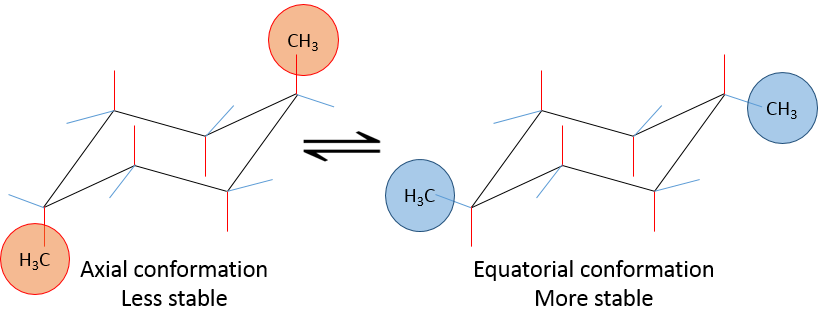If the second methyl group was on an axial spot, the two conformers are equivalents (the axial methyl becomes equatorial and vice versa). The total energetic advantage of one of the conformer is indeed 7.1kJ/mol-7.1kJ/mol=0kJ/mol.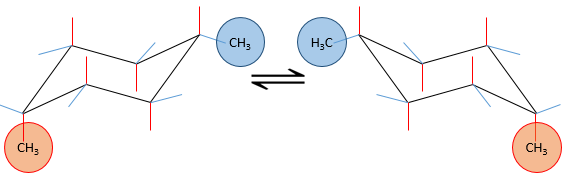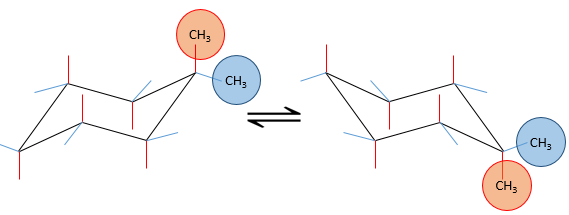If the second group was a chlorine atom instead of a methyl group, we proceed the same way to know which conformer is favoured. A single chlorine group stabilizes the equatorial conformation by 2.2kJ/mol. If both substituents were equatorial, the total energetic advantage of the equatorial conformer is 7.1kJ/mol (from CH3) +2.2kJmol (from Cl)=9.3kJ/mol. This molecule is thus more stable than the methylcyclohexane.

If the chlorine atom was axial, the total energetic advantage of the conformer wherein the methyl is equatorial is 7.1kJ/mol-2.2kJ/mol=4.9kJ/mol. The conformer with an equatorial methyl is still more stable than the other conformer but less than the methylcyclohexane.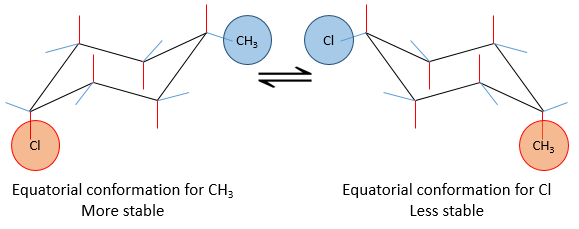Note that other interactions may affect the stability of the conformers. When the substituents are on other spots than 1-4, interactions (as steric hindrance, repulsion,…) may decrease or increase the energetic advantage of one conformer on the other one.

Polycyclic alkanes

Molecules are not limited to one cycle. Several cycles may share carbons. A molecule composed of two hexanes sharing two carbons is called decaline and exists in a trans and a cis form. Cycles do not have to be the same size and a cyclohexane can merge with a cyclopentane.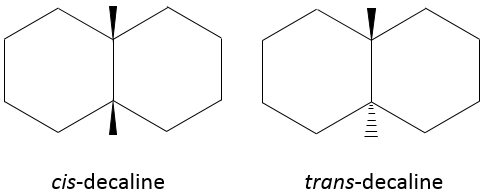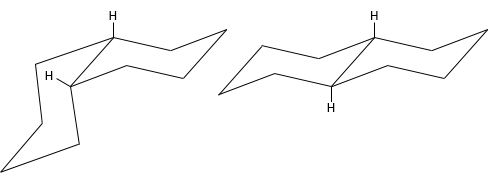Two cycles may also be slotted one into each other. This leads to bridged cycles. The norborane is a bridged cyclohexane or two cyclopentane sharing 3 carbons. Carbons wearing the bridge are called bridgeheads.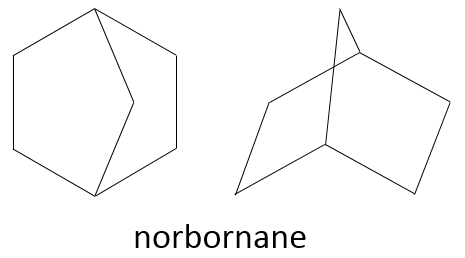Polycyclic alkanes reduce the freedom of move of the molecule but it seems that there is no limit to the cycle tension that hydrocarbures can endure, as one can think from the bicyclobutane.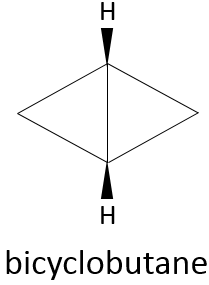Polycycles with cycles of few carbons (3-4) are in general not naturally produced but may be made through synthesis. All types of carbon skeletons have been made in laboratories. They can have an interest as explosives if they wear nitro croups thanks to their important cycle tension.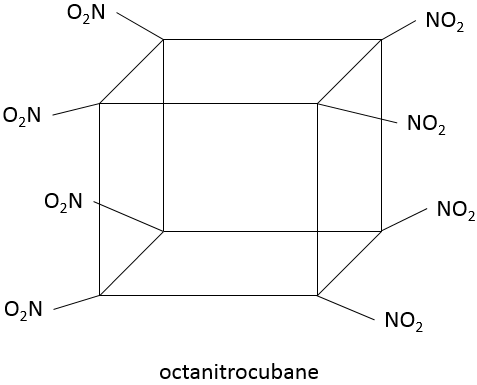Polycycles of larger cycles are often found in natural compounds, giving specific odors, parfums, colors, or very specific roles as do have hormones.